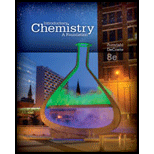# Consider a reaction represented by the following balanced equation :math&gt; 2 A + 3 B → C + 4 D u find that it requires equal masses of A and B so that there are no reactants left over. Which of the following is true? Justify your choice. l type='a'&gt; The molar mass of A must be greater than the molar mass of B. i&gt;The molar mass of A must be less than the molar mass of B. i&gt;The molar mass of A must be the same as the molar mass of B.BuyFind

### Introductory Chemistry: A Foundati...

8th Edition
Steven S. Zumdahl + 1 other
Publisher: Cengage Learning
ISBN: 9781285199030BuyFind

### Introductory Chemistry: A Foundati...

8th Edition
Steven S. Zumdahl + 1 other
Publisher: Cengage Learning
ISBN: 9781285199030

#### Solutions

Chapter 9, Problem 13ALQ
Textbook Problem

## Expert Solution

### Want to see the full answer?

Check out a sample textbook solution.

### Want to see this answer and more?

Experts are waiting 24/7 to provide step-by-step solutions in as fast as 30 minutes!*

*Response times vary by subject and question complexity. Median response time is 34 minutes and may be longer for new subjects.© 2021 bartleby. All Rights Reserved.